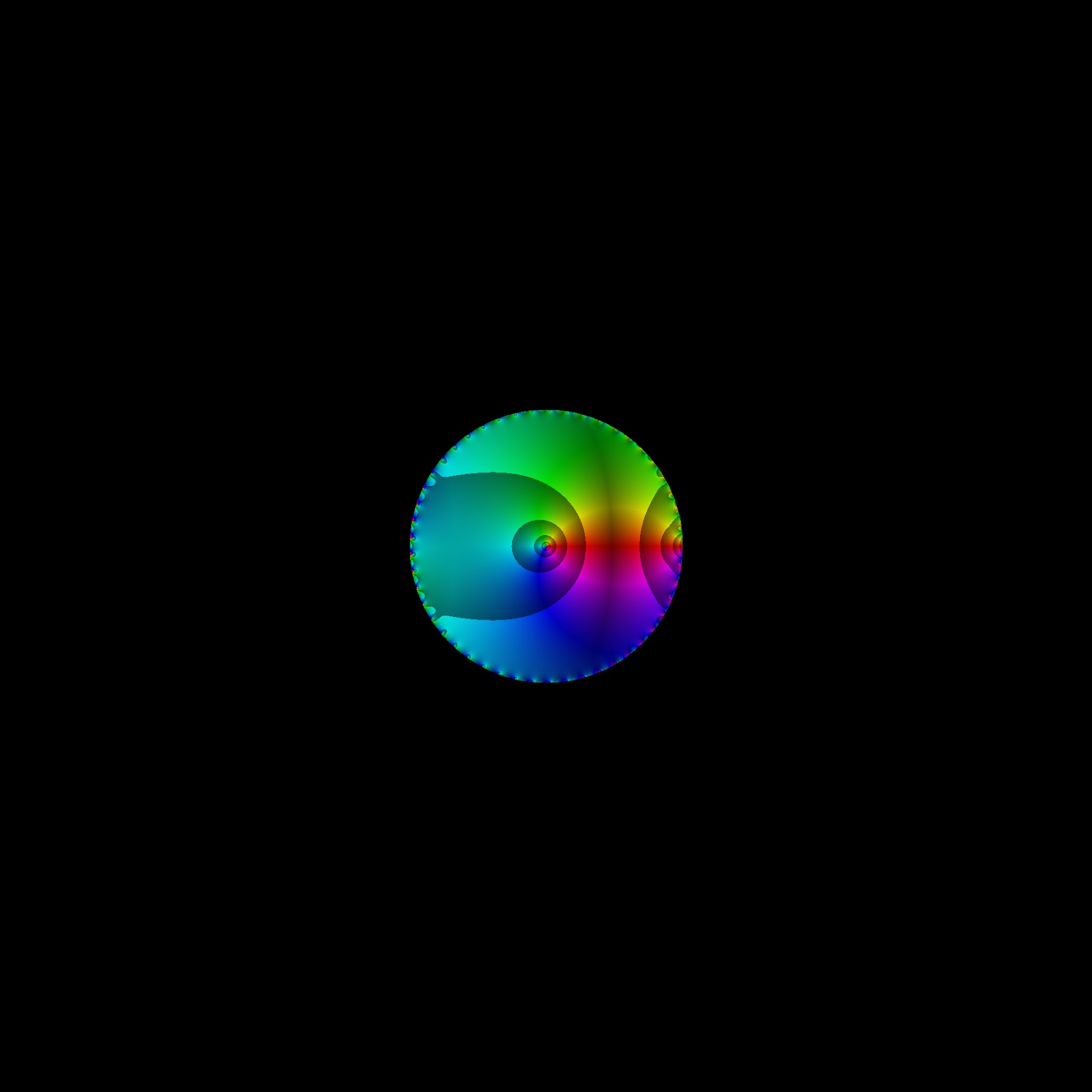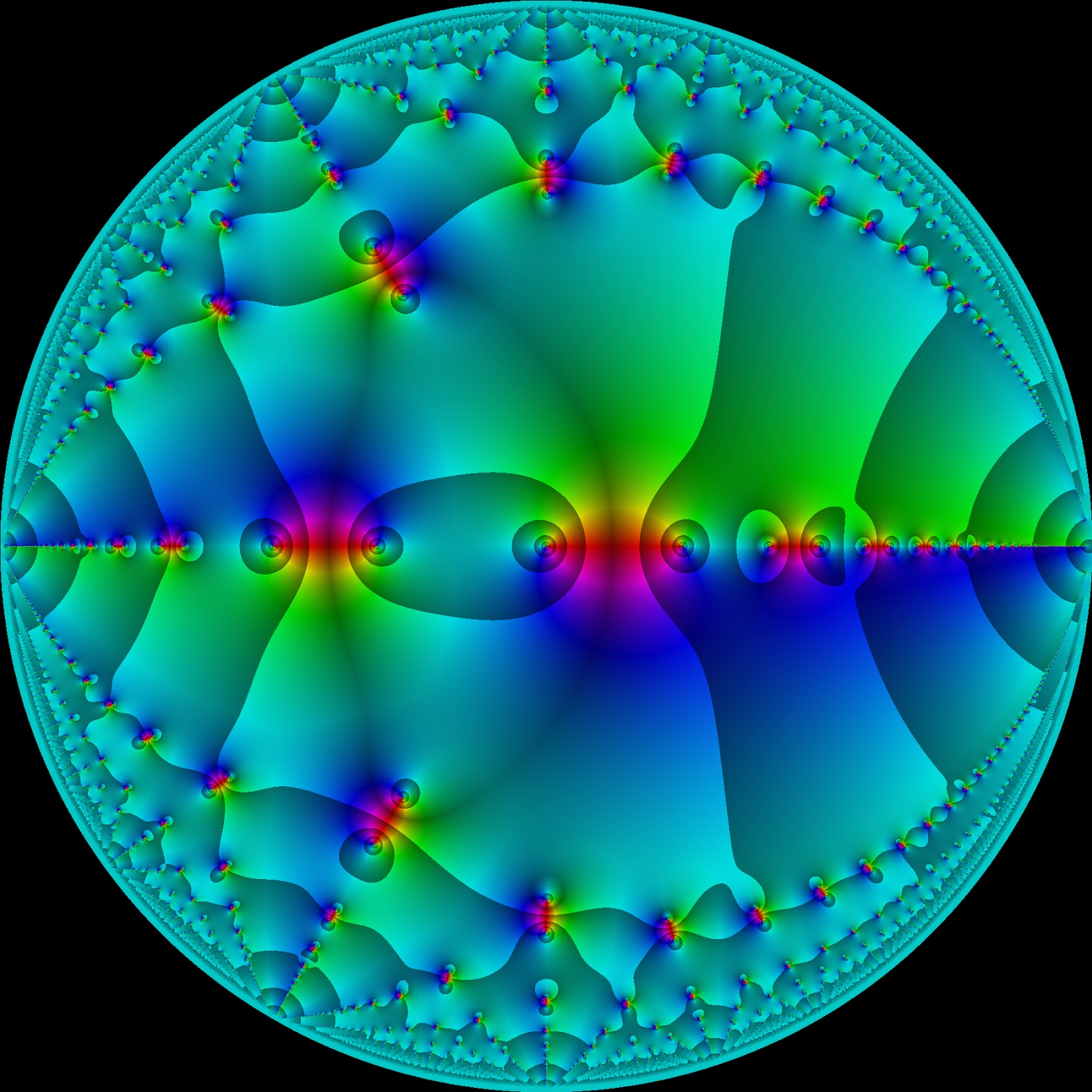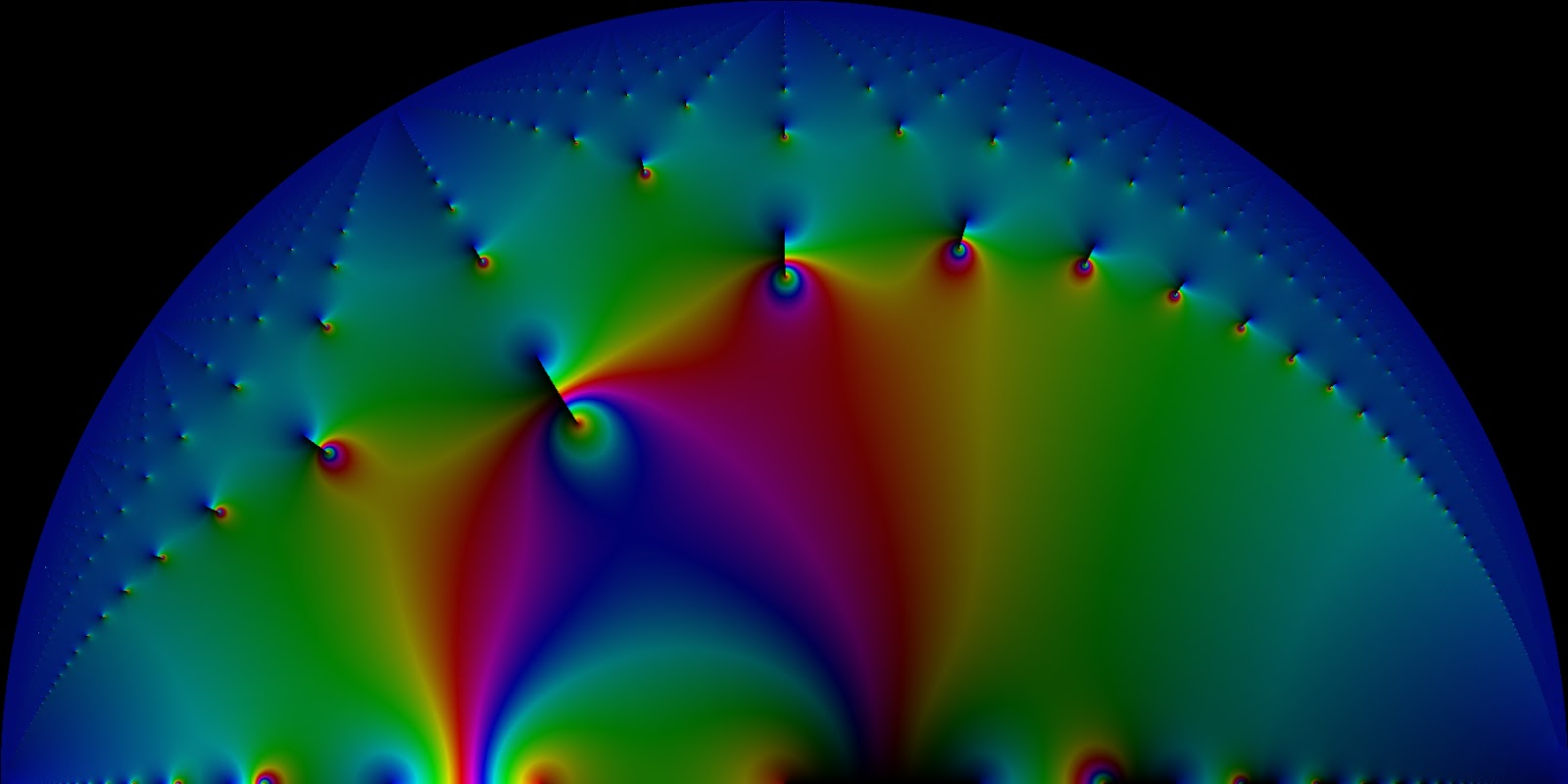# Analytic continuation of a lambert series $f(z)=\sum_{n=1}^\infty \frac{2^n z^n}{1-(z/2)^n}$?

Let's define a function for complex $$z$$ :

$$f(z)=\sum_{n=1}^\infty \frac{2^n z^n}{1-(z/2)^n}$$

Lambert series often have an analytic continuation and can even be entire functions so the poles can be removable.

As typical example we have $$\sum_{n=1}^\infty \frac{\mu(n) z^n}{1-z^n}=z$$ where $$\mu(n)$$ is the moebius function.

For our function $$f(z)$$ we have a dense set of poles with $$|z| = 2$$ although they might be removable.

But our function starts to diverge as soon as $$|z|>\frac{1}{2}$$.

So the question is how to do analytic continuation of $$f(z)$$.

Perhaps

$$f(z)=\sum_{n=1}^\infty \frac{2^n z^n}{1-(z/2)^n}=\sum_{n=1}^\infty \frac{4^n (z/2)^n}{1-(z/2)^n}$$

can help ?

We could maybe use $$4^n=\sum_{m=0}^\infty \frac{\ln(4)^m n^m}{m!}$$ and use a double sum ?

We have a closed form of $$f(z,t) = \sum_{n=1}^\infty \frac{n^t z^n}{1-z^n}$$ for every positive integer $$t$$ afterall in term of taylor series with coefficients from the generalized divisors function.

But I am not sure if that helps.

We could try to take taylor series expansions at various points but Im not sure how to find the radius.

Can we compute $$f(3)$$ ?? Does $$f(z)$$ have any natural boundaries, and if so where are they ? Is $$f(z)$$ a meromorphic function ?

Does it help to define $$1 + 2 + 4 + 8 + 16 + ... = -1$$ ? What summability methods should we or could we use here ?

• if $z=re^{it}$ st $e^{ikt}=1, r<1/2$ then the partial sums of $f$ are $\sum c_m(2r)^m+r_m$ with $c_m >\delta >0$ and $r_m$ uniformly bounded, so $|f(z)| \to \infty, r \to 1/2$ hence $f$ has $|z|=1/2$ as natural boundary Mar 7 at 23:29
• f has $|z| = 2$ as its natural boundary. It has a meromorphic continuation up to that natural boundary, where the poles accumulate. Beyond that boundary, there is a "natural" extension (up to some simple factor). Mar 8 at 0:47
• @Caleb what is $f(w/2)$ when $w$ is an arbitrary root of unity? If $f$ would be continued meromorphically to $|z|=2$, the poles cannot accumulate so in particular there is a $|c|=1/2$ for which $f$ is analytic in a neighborhood of $c$ but then there would be points $w/2, w$ root of unity at which $f$ is analytic and I believe $|f(rw/2)| \to \infty, r \to 1$ which precludes that Mar 8 at 1:47
• @Conrad I think you're looking at $\frac{1}{1-(2z)^n}$. For $\frac{1}{1-(z/2)^n}$, the problems only start to occur when $|z| = 2$, since then when $z$ is 2 times a root of unity the poles start to accumulate. Mar 8 at 2:42
• The numerator is $(2z)^{2n}$ so doesn't converge to zero when $|z| \ge 1/2$ Mar 8 at 2:46

I'll come back and write a more formal answer later. For now, I'll just give a brief argument and then show some pretty pictures ;)

Using the geometric series, we have that (for $$|x|<2$$) $$\sum_{n=1}^\infty \frac{2^nx^n}{1-(\frac{x}{2})^n} = \sum_{n=1}^\infty 2^nx^n \sum_{k=0}^\infty \frac{x^{nk}}{2^{nk}}$$ The sum absolutely converges for $$|x|<1/2$$, so we can switch the order of summation to obtain $$\sum_{n=1}^\infty 2^nx^n \sum_{k=0}^\infty \frac{x^{nk}}{2^{nk}}= \sum_{k=0}^\infty \sum_{n=1}^\infty \frac{x^{n(k+1)}}{2^{n(k-1)}}$$ Now that the inner sum coverges when $$|x|<2$$, and we get $$\sum_{k=0}^\infty \sum_{n=1}^\infty \frac{x^{n(k+1)}}{2^{n(k-1)}} = \sum_{k=0}^\infty \left(\frac{1}{1-\frac{x^{k+1}}{2^{k-1}}}-1\right)$$ Define $$f(z) =\sum_{k=0}^\infty \left(\frac{1}{1-\frac{z^{k+1}}{2^{k-1}}}-1\right)$$. Then $$f(z)$$ is meromorphic for $$|z|<2$$ and agrees with the original series for $$|z|<1/2$$. By the identity theorem, it is an analytical continuation up to $$|z|<2$$. At that point, it has a natural boundary.

Now to the interesting part-- pictures!This is a graph of the original function, before continuation. And this is what the continuation looks like:There are some really striking patterns in the locations of the zeroes and poles. For instance, if I change some of the domain coloring properties, I get this beautiful picture of the function (where only the top half is graphed, since the rest is symmetric)Now, the real interesting question is 'Can we continue this function beyond its natural boundary? What does the function look like there?' I don't know the answer to these questions, but I hope to make some progress on them soon.

## Explanation of the Pictures

Picture 1 and Picture 2 share the same coloring method. In HSB (Hue, Saturation, Brightness), the function I'm using is (with 255 as the highest value) $$F(re^{i \theta}) = \left[ 255\frac{\theta}{2\pi},250,125 + mod(155|\ln(r)|,255) \right]$$ So, the hue corresponds to the angle. The brightness corresponds to the log of the size. The breaks in color you appear to see come from the mod(x,255) term. This means the color resets backward when it becomes too large. This makes it easy to detect poles and zeroes, since their will be lots of these breaks close together near poles and zeroes, since there the log of the size becomes infinite.

In the second picture, I make the hue correspond to the angle. This is why you see those cuts-- the angle isn't a continuous function. In particular, if you go around a circle, the angle goes from $$0$$ to $$2 \pi$$, but then jumps back to $$0$$. So the lines correspond to points where the angle is jumping from $$0$$ to $$2 \pi$$. Thus, along every cut, the function is purely real with a positive real part.

The cuts connect together the poles and zeroes in this function. In particular, if I draw the poles in white, then I obtain this graph:(Ignore the white near the boundary, that is just because it becomes too hard to compute the function accurately there). I think that's pretty interesting, the poles and zeroes seem to come in pairs, and those pairs seem to be connected

• What are the short straight lines in the last picture ?? that does not look analytic ? All the poles are at |z| = 2 right ? What is the striking patterns of poles and zero's ? Is there a functional equation for the function or for the zero's ? This looks like many lambert series at first ? Such as j-invariant. I did notice that the second layer contains about twice as many zeros as the first layer. And every zero seems to a member of 3 " straight" lines on a sphere. I think this 2 and 3 come from 2^n and z/2, suggesting that if we plot the 5 analogue we get similar patters but with 5 and 4.
– mick
Mar 8 at 23:34
• @mick I'll append an explanation of the colorings to my answer Mar 9 at 2:17
• oh wow so the lines connect zeros and poles ! I did not expect the poles so far from the boundary. Not even after I have seen your resummation. Maybe I am missing something.
– mick
Mar 9 at 21:59
• @mick The poles happen when $1- \frac{x^{k+1}}{2^{k-1}}$, so this means that $1 = 4 (x/2)^{k+1}, 2\cdot \sqrt[k+1]{\frac{1}{4}}= x$. So, this is less than 2 in absolute value, and as you take n to become large, these poles become closer and in absolute value to 2, but also get more and more dense. Mar 10 at 0:55
• Right right sorry +1
– mick
Mar 10 at 21:05

This is not an answer(yet?) but more of a comment and in particular aimed at Caleb.

To extend beyond Caleb answer for $$2<|z|$$ I talked to my mentor tommy and he mentioned the generalized q-gamma function. This might work.

Here is the basic idea or at least my interpretation.

Say $$x=10$$.

Then the sum is

$$f(10) = \sum \frac{20^n}{1 - 5^n}$$

Now lets use taylor series , say

$$g(x) = \sum \frac{x^n}{1 - 5^n}$$

This implies

$$g(x) - g(5x) = \frac{1}{1-x}$$

And that has the solution for a suitable constant $$C$$ :

$$g(x) - C = \frac{\psi_5(\frac{\ln(x)}{\ln(5)}) - \psi_5(1)}{\ln(5)} - \frac{\ln(x)}{\ln(5)}$$

Where $$\psi$$ is the q-gamma function.

The q-gamma function satisfies recursion equations and reflection equations so this might just work.

So

$$f(10) = g(20) = C + \frac{\psi_5(\frac{\ln(20)}{\ln(5)}) - \psi_5(1)}{\ln(5)} - \frac{\ln(20)}{\ln(5)}$$

edit

ps :

See

https://en.wikipedia.org/wiki/Q-gamma_function

where many formulas are explained.

• @Caleb are you reading this ?
– mick
Apr 8 at 20:01
• Extending beyond a natural boundary is hard to do rigorously. However, one thing to consider is that $$\sum_{n=1}^\infty \frac{2^n x^n}{1-\frac{1}{\alpha} (\frac{x}{2})^n}$$ Is meromorphically continued to $$\sum_{k=0}^\infty \frac{1}{\alpha^n} ( \frac{1}{\frac{x^{k+1}}{2^{k-1}} }-1)$$ This object converges even when $|z|>2$, so it's a natural sort of extension. Is it the right extension? James from the Tetration Forum wrote a paper that suggests the answer is yes. But even if we accept that it's not clear we are allowed to take the limit as $\alpha \to 1$... Apr 9 at 22:47
• I don't know much about the q-gamma function, or how to compute it. But, if you get some computations on the value that the q-gamma function computes at some different points, I could compare to the method of extension I mentioned in my last comment. For instance, what would the q-gamma extension evaluate f(3) as? What about f(-2-2i)? Apr 9 at 22:49
• @CalebBriggs from computing two values as given in my answer, we get the constant value $C$ so we should get a closed form. I bet $C$ is even a simple number. The formula given in the wiki that relates q-gamma(q) with q-gamma(1/q) is probably the one we need. Also notice that for the alpha sum this is also a geometric series so analytic continuation can be done there. For the case alpha = 1 we get the q-gamma reflection I just mentioned. Well that is the basic idea. I wonder if this method agrees with -1/2 for$1+1+1+1+...$ since this can clearly be seperated here
– mick
Apr 10 at 11:17
• What makes the q-gamma special is that it satifies a reflection and iteration equation in an analytic way , making it a uniqueness condition and logical.
– mick
Apr 10 at 11:25